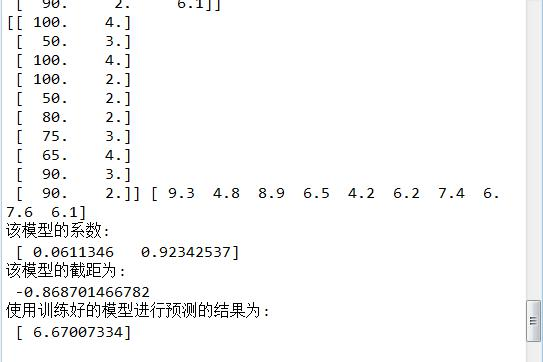# 机器学习之多变量回归模型（一）

#### 代码


#这里讲一下关于多变量回归模型的预测问题，这里涉及csv文件的读取
#以及使用sklearn库中的linear-model进行训练的

from numpy import genfromtxt
import numpy as np
import csv
datapath=r'F:\workspace_python\py_mechinelearning\20180623\delivery.csv'
data=genfromtxt(datapath,delimiter=',')
print(data)
X=data[:,:-1]
Y=data[:,-1]
print(X,Y)
LR=linear_model.LinearRegression()  #调用sklearn中的linear_model中的LinearReression模型
LR.fit(X,Y) #使用该模型进行训练X,Y 训练集上训练模型
print("该模型的系数:\n",LR.coef_)
print("该模型的截距为:\n",LR.intercept_)

X_test=[[78,3]]
print("使用训练好的模型进行预测的结果为:\n",LR.predict(X_test))


#### 结果